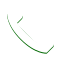# How to approach mathematics for NDA

Mathematics a fear for most of us, BUT when it comes to defence forces maths is one of the most important subjects for the written exam NDA, CDSE, AFCAT and INET . According to the recent trends, the questions asked from maths are quite simple after apply some concept , the exam conducted by the UPSC are based on the concept one who knows the concept can solve all the questions .

Role of maths

1.Maths is highly scoring subject and more the marks more the chances to qualify for the dream force. (ARMY, NAVY,AIRFORCE)

2. Maths  also plays important role during OIR test in 1st stage of SSB.

3. Different types of questions increases analytical ability and help in developing a problem solving attitude in life.

4. Maths is quite different from general knowledge as it is the subject that doesn’t have current affairs , all the things remain the same.

Important parts of mathematics which are required for different exams starting from CDS

1.) Arithmetics at comprises of simple aptitude based questions from the topics like time and work, ratio and proportion, average, cost price and selling price discount simple interest compound interest unitary methods. this part is of 40 marks.

2.) Geometry this part covers the topics like line and angles, triangles, similarities of triangle and different centres of triangle, circle, quadrilaterals, polygons. From this part nearly 20 to 25 marks questions will be asked in the examination.

3.) Algebra this part covers the topics like identity based questions polynomials HCF and LCM of polynomials line representation of polynomials solution of polynomials and other questions based on remainder theorem of polynomials. From this part nearly 20 marks questions are asked in the examination.

4.) Trigonometry from this part question based on different ratios and their identities are asked, questions based on zero degree to 90 degree of angle , maxima and minima of trigonometric ratios and height and distance based questions also asked. From this part generally 20-25 questions are asked.

5.) Statistics it includes the topics like grouped data, histogram, bar graph and pie chart. From this topic generally 5 questions are asked in the examination.

for AFCAT and INET questions from the arithmetic part is asked each question carrying 3 marks and the total of 18 questions is asked and hence this part generally is a 3 multiply 18 gives 54 marks.

How to prepare for maths exam?

1. Know the syllabus of the exam
2. Read each and every topic with the concept and practice at least 100 questions of each topic / chapter
3. For AFCAT focus on arithmetics  , for CDSE focus on advance maths.
4. After doing all the chapters start solving some mocks .
5. Most important practice each and every previous year question paper.
6. Practice according to real exam timings to make the mind habitual of working at exam timings like if anyone is preparing for CDSE exam in real  maths exam is from 3pm to 5pm so while practicing solve the mocks and previous year questions from 3-5 pm.
(NAVY -AA(2012),NDA 1(2012),NDA 2(2012), CDSE 1&2(17,18), CDSE 2019 (1), AFCAT 1 & 2 (2016(2),17,18,19))

## Our Course

B-277, Ground Floor near Ramphal Chowk,
opp. IGNOU Center,
Sec-7.Dwarka-75
New Delhi

For Enquiry:- 9354048476, 9313223768Call Me